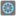## Question related to missionEven the Last

I can get the solution on spyder on desktop but here it does not work!

```evenss = []
result=[]
def checkio(array: list) -> int:
for i in range(len(array)):
#print(i)
if array[i] %2==0:
evenss.append(array[i])
result=(sum(evenss))*(array[-1])
print(result)
return result

#These "asserts" using only for self-checking and not necessary for auto-testing
if __name__ == '__main__':
print('Example:')
print(checkio([0, 1, 2, 3, 4, 5]))

assert checkio([0, 1, 2, 3, 4, 5]) == 30, "(0+2+4)*5=30"
assert checkio([1, 3, 5]) == 30, "(1+5)*5=30"
assert checkio() == 36, "(6)*6=36"
assert checkio([]) == 0, "An empty array = 0"
print("Coding complete? Click 'Check' to review your tests and earn cool rewards!")
```7